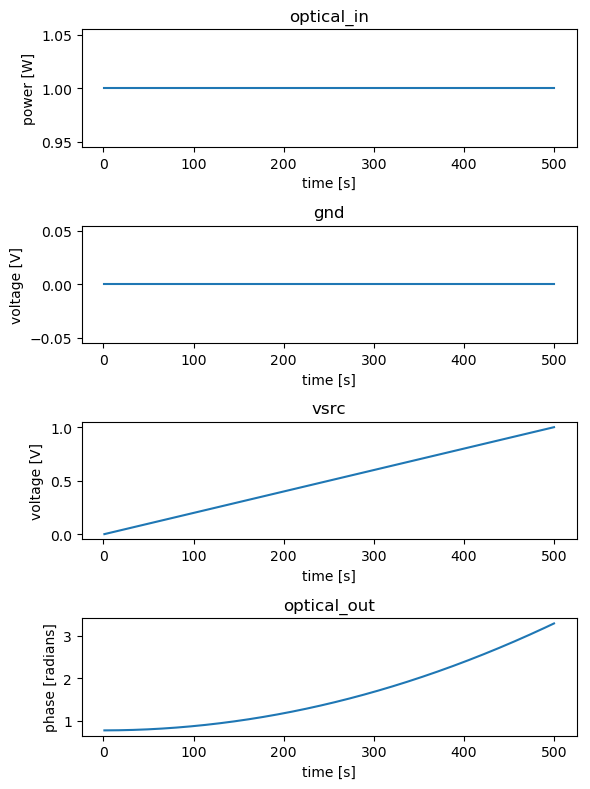# Heaters¶

SiFab contains one heater.

## HeaterWaveguide¶

This component consists of a waveguide with a heater layer on each side, drawn on the HT layer. It is used to change the phase of the light travelling through the waveguide by exploiting the thermo-optic effect in silicon. At the beginning and at the end of the waveguide, metal vias are used to connect the heater metal to a metal contact pad on the M1 layer.

The following parameters can be changed:

• contact_pitch: Contact pitch
• heater_width: Width of the heater layer
• heater_offset: Offset of the heaters from the center
• m1_width: Width of the M1 section
• length_via_section: Length of the via section
• via_pitch: Via to Via pitch
from si_fab import all as pdk
from ipkiss3 import all as i3

# Heater
ht = pdk.HeatedWaveguide(contact_pitch=0.6,
heater_width=0.6,
heater_offset=1.0,
m1_width=1.0,
length_via_section=3.0,
via_pitch=1.0,)
ht_lv = ht.Layout(shape=[(0.0, 0.0), (10.0, 0.0)])
ht_lv.visualize(annotate=True)

xs = ht_lv.cross_section(cross_section_path=i3.Shape([(1.0, -8.0), (1.0, 8.0)]))
xs.visualize()

ht = pdk.HeatedWaveguide()
start_angle=280,
end_angle=260))
ht_lv.visualize(annotate=True)### Model and simulation recipes¶

The phase of the waveguide is calculated using the effective index ($$n_{eff}$$) of the trace template (trace_template) used to draw the waveguide. The model of the heater adds a phase modulation to the phase of the waveguide that is calculated as follows:

$\Delta \Phi = \frac{\pi V_d^2}{P_{\pi\square}}\frac{W}{L}$

where

• $$L$$ and $$W$$ are the length and width of the heater, respectively;
• $$V_d$$ is the voltage drop over the phase shifter length;
• $$P_{\pi\square}$$ is the power required on a square of heater material with a resistance of 1 $$\Omega / \square$$ to achieve a $$\pi$$ phase shift.

The power dissipated over the heater to achieve a $$\pi$$ phase shift can be calculated as

$P_{\pi} = \frac{P_{\pi\square}}{R_{SH}}$

where $$R_{SH}$$ is the sheet resistance of the heater metal.

Using the simulation recipe simulate_heater one can easily check the phase shift caused by a voltage sweep from $$0$$ to $$1$$, as well as the associated current density. The sheet resistance $$R_{SH} = 0.5$$ $$\Omega / \square$$ and maximum current density $$j_m=0.05$$ $$A/ \mu m ^2$$ are stored in the SiFab PDK and can be considered fixed.

from si_fab import all as pdk
from si_fab.components.heater.simulate.simulate import simulate_heater
from si_fab.components.heater.pcell.cell import r_sheet, j_max
import pylab as plt
import numpy as np
import os

# Phase Shifter
name = "heater_sweep"
results_array = []
length = 100
ht = pdk.HeatedWaveguide(contact_pitch=0.6,
heater_width=0.6,
heater_offset=1.0,
m1_width=1.0,
length_via_section=3.0,
via_pitch=1.0,)
ht_lv = ht.Layout(shape=[(0.0, 0.0), (length, 0.0)])

p_pi = 30e-3  # W
p_pi_sq = r_sheet * p_pi
ht.CircuitModel(p_pi_sq=p_pi_sq)

results = simulate_heater(cell=ht,
v_bias_start=0,
v_bias_end=1,
nsteps=500,
center_wavelength=1.5,
simulate_sources=True,
simulate_circuit=True,
debug=False)
times = results["timesteps"]
results_array.append(results)

def phase_unwrap_normalize(results):
unwrapped = np.unwrap(np.angle(results))
return unwrapped - unwrapped

outputs = ["in", "elec1", "elec2", "out"]
process = [np.real, np.real, np.real, phase_unwrap_normalize]
titles = ["in", "elec1", "elec2", "out phase"]
ylabels = ["", "V", "V", "rad"]
fig, axs = plt.subplots(nrows=len(outputs), ncols=1, figsize=(6, 8))

for cnt, (f, pn, title, ylabel) in enumerate(zip(process, outputs, titles, ylabels)):
axs[cnt].set_title(title)
axs[cnt].plot(times, f(results[pn]), label="length: {}".format(length))
axs[cnt].set_ylabel(ylabel)

plt.tight_layout()
fig.savefig(os.path.join("{}.png".format(name)), bbox_inches='tight')